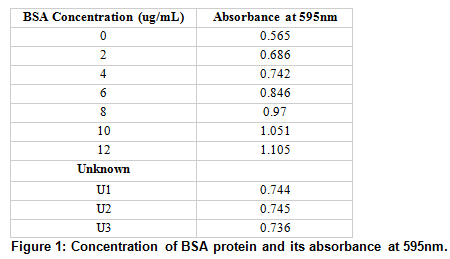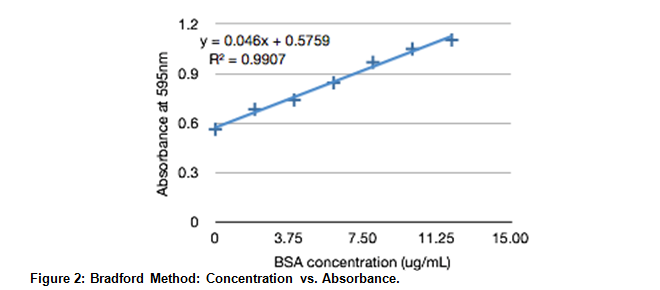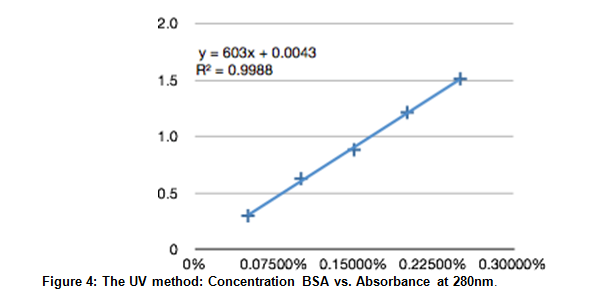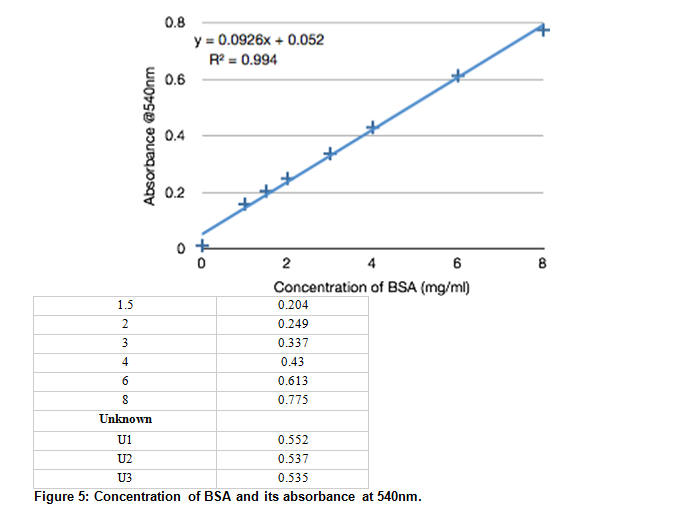# Measuring Protein Concentration

By: Amanda Campbell

Introduction:

This experiment was performed in order to explore three different methods of measuring protein concentration: the Bradford method, the UV method, and the Biuret method. All three methods are quick methods to determine the concentration of protein in a solution, although none can determine the concentration of a specific protein in a solution of proteins.

The Bradford method uses a blue dye, Coomassie Brilliant Blue G-250, which binds to positively charged side groups on amino acids. The anionic form of the dye has a peak absorbance at 595nm, so the amount of dye bound to the amino acid can be found by reading the absorbance at that wavelength.

The UV method makes use of the actual proteins absorption in the ultraviolet spectrum. This method is fast, easy to automate, and nondestructive, which makes it ideal for determining protein concentrations. The downside of this method is the solution being analyzed would have to be relatively pure as other compounds also absorb at 280nm.

The Biuret method uses a Biuret reagent which reacts with proteins to form a Cu (II) ion with four nitrogens. This ion has a purple color, which has a peak absorption at 540nm. The intensity of the color is indicative of protein concentration. This method requires at least 1-20mg of protein, a relatively large amount. Also, false positives can be given if there is any ammonia or ammonium ions in the solution.

All of the methods described have limitations, which is why all three are demonstrated here. Each method is best in different situations.

The methods described here all relate absorption at a certain wavelength to concentration of protein. This relationship is described by the Beer-Lambert law which states A = E*l*C where A is absorbance, E is the molar extinction coefficient, l is the path length is cm, and C is molar concentration.  The absorbance of a protein is a physical property that belongs to it, just like a melting point or freezing point. The molar extinction coefficient is a number determined by the amount of tryptophan and tyrosine residues that are in the protein. So, because all values except concentration are determined by the physical properties of the protein being analyzed, you can easily find the concentration using the absorbance: C = A/E*l .

Methods:

Directions were followed as laid out in Chem 431 Lab Manual (pp 14-23). The only changes were made during the Bradford Method. The Bradford Reagent was added to each microcentrifuge tube in 1 minute intervals to insure each sample was exposed for the same amount of time.

Results:The BSA protein concentration and its absorbance are shown, along with the sample of unknown concentration (sample #32) and its absorbance, taken three times.The absorbance at 595nm is plotted against protein concentrations. A trend line based on the collected data is given at y=0.046x + 0.5795 with a .9907 correlation.

Using the linear approximation found using experimental data, the concentration of the unknown (sample 32) was found.

.742 = 0.046x + 0.5795

x = 3.53 ug/mL

Part II: The Ultra Violet Absorption Method

 BSA Concentration Absorbance @280nm 0.05% 0.3 0.10% 0.629 0.15% 0.885 0.20% 1.216 0.25% 1.514 Unknown 1 0.909 2 0.914 3 0.912

Figure 3: Concentration of BSA and its absorbance at 280nm. The BSA protein concentration and its absorbance are shown, along with the sample of unknown concentration (sample #29) and its absorbance, taken three times.The absorbance at 280nm is plotted against protein concentrations. A trend line based on the collected data is given at y=603x + 0.0043 with a .9988 correlation.

Using the linear approximation found using experimental data, the concentration of the unknown (sample 29) was found.

.912 = 603x + .0043

x = 15%

A sample of pure BSA protein was analyzed and had a 260/280 ratio of .623;  A sample of pure DNA was analyzed and had a 260/280 ratio of 1.643.

Part III: The Biuret Method

 BSA Concentration (mg/ml) Absorbance at 540nm 0 0.011 1 0.158The BSA protein concentration and its absorbance are shown, along with the sample of unknown concentration (sample #47) and its absorbance, taken three times.

Figure 6: Biuret Method: Concentration vs. Absorbance. The absorbance at 540nm is plotted against protein concentrations. A trend line based on the collected data is given at y=0.0926x + 0.052 with a .994 correlation.

Using the linear approximation found using experimental data, the concentration of the unknown (sample 47) was found.

.541 = .0926x + .052

x = 5.28 mg/mL

Discussion:

The results for the lab were both relatively accurate a precise. The correlation factors for each method were all above .99 which indicates precise readings. The comparison of known 260/280 data and experimentally discovered values were close, which leads to the conclusion of accurate results. Because the results were both accurate and precise lead to confidence in the results of this lab.

Accepted values for pure protein 260/280 absorbance ratio is about .5 and the value found in this lab was .623. Accepted values for pure DNA 260/280 absorbance ratio is about 1.6 and the value found in this lab was 1.643.

Using the linear fit line found in this lab using the UV method, a 1% solution of BSA should absorb 6.03 at 280nm. Modern spectrophotometers can generally only report to 3.0 absorbance units, so it would be impossible to find this value experimentally.

The absorbance for a 1M BSA solution is 43,824 M−1cm−1. The molar extinction coefficient depends on the number of Tryptophan and Tyrosine residues in the the protein, so a protein that weighs 66kD could have a different number of Tryptophan and Tyrosine residues and therefore a different molar extinction coefficient.

References:

Kruger, Nicholas J. “The Bradford Method for Protein Quantitation.” Methods in Molecular Biology 32         (1994): 9-15. Print.

Arnaldo Yoshiteru Kuaye, An ultraviolet spectrophotometric method to determine milk protein content in          alkaline medium, Food Chemistry, Volume 49, Issue 2, 1994, Pages 207-2. Print.

Christopher Watters, A one-step biuret assay for protein in the presence of detergent, Analytical          Biochemistry, Volume 88, Issue 2, 1 August 1978, Pages 695-698. Print.

Bradford MM (1976) A rapid and sensitive method for the quantization of microgram quantities of protein      utilizing the principle of protein-dye binding. Anal Biochem 72: 248-54. Print.

Chem 431 Lab Manual 2013 Central Washington University. 10-23. Print.

• Share